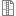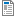# 17ABBUSS - Introduction to Signals and Systems

Code Completion Credits Range Language
17ABBUSS Z,ZK 4 2P+2C English
Lecturer:
Tutor:
Supervisor:
Department of Biomedical Informatics
Synopsis:

To introduce students to basics of theory of signals and systems. To explain main principles on applications from biology and medicine. To become acquainted with basic mutual relations in computer laboratories by means of MATLAB.

Requirements:

Credit inclusion: Taking two tests with at least 50 % sucess each. First test is dedicated to signals and second to systems. Knowledge of practical use of Matlab.

Exam: Written and oral.

Syllabus of lectures:

1. Systems and signals. Practical tasks and motivation. Biosignal processing (filtration). Examples of physiological models. ( pages 1-3)

2. Signals. Basic terms and definitions. Continuous signals. Basic operations with signals. Mathematic models of basic continuous signals. Periodic signals. Unrepeated signals. ( pages 6-16)

3. Decomposition of continuous periodical signals to single harmonic components. Fourier transformation. Features. ( pages 153-189)

4. Discrete signals. Sampling. Basic operations with discrete signals. Mathematic models of basic discrete signals. Decomposition of discrete periodical signals to single harmonic components. ( pages 297-323)

5. Fourier transformation with discrete time. Discrete Fourier transformation. Fast Fourier Transform. ( pages 339-345)

6. Convolution. Definitiona and basic equations. Geometric im,portance of convolution.Billinear transformation used to prepare digital filter IIR. ( pages 101-114)

7. Systems. Basic attributes of systems. Technical and biological systems. Systems and their description. Continuous systems. Inner and outer description. MIMO systems. ( pages 57-63, 656-660)

8. Forms of outer description of continuous linear system - differential equation, frequnce transfer characteristics, pole and null location, time characteristics. ( pages 203-208, 394-407)

9. Systems with discrete time. Forms of outer description of linear system - difference equation, transfer functions, frequence characteristics, pole and null location, time characteristics. ( pages 581-597)

10. Basic phenomenons in systems - investigation of initial state influence and exploration of output influence. ( pages 509-513)

11. Stability. Basic terms and definitions. Stability of forced movement. Stabiluity with respect to initial state. Criteria of stability. ( pages 527-543)

12. System connecting. Serial and parallel ordering. Feedback (closed-loop system). Feedback control. Features of feedback. General connection of systems - method of consecutive modifications. Mason's rule. ( pages 436-443)

13. Exam.

Syllabus of tutorials:

1. Programming environment MATLAB - introduction, possibilities, usage, generating, reading, storing and displaying some types of experimental signals (harmonic signal, unit impulse, unit jump). Principle of sampling.

2. Programming environment MATLAB - generating, reading, storing and displaying some types of experimental signals (harmonic signal, unit Fourier transform impulse, unit jump). Principle of sampling.

3. Fourier transform, Fourier series. Amplitude Spectrum, phases spectrum.

4. Continuous and Discrete convolution, practice of manual and computer calculation, importance of convolution for signal filtering.

5. Billinear transformation using to prepare digital filter IIR.

6. control test.

7. Dynamic properties of continuous systems. External description (differential equations, transfer function, frequency transfer, frequency response, distribution of zeros and poles).

8. Dynamic properties of continuous systems. Practicing procedures to analyze external description. Systems interconnection (serial, parallel, feedback). The principle of feedback control. Validation in MATLAB environment.

9. Impulse and transient characteristics. Z-transformation. Comparison of continuous and discrete systems.

10. Stability of systems. Aperiodicity and accuracy of regulation. Method to achieve it.

11. Proportional plus Integral plus derivative control (PID)

12. Setup methods of regulator parameters according to stability conditions.

13. control test.

Study Objective:

The goal of the subject is to introduce students to basics of signals, thier processing and manipulation in time and frequency domain. The subjects focuses on Fourier analysis. The second part of the subject is dedicated to basics of systems, their description and features

Study materials:

B.P.Lathi. Linear Systems and Signals, Oxford, Oxford University Press, ISBN 0-19-515129-1.

Kamen, E.W. Heck B.S. Fundamentals of signals and Systems using the Web and Matlab.Prentice Hall, ISBN 0-13-017293-6.

Note:
The course is a part of the following study plans:
##### Materiály ke stažení:

Cvičení:
PřílohaVelikostdesign IIR filter by Billinear transformation135.29 KBFourier_series_transform1.75 KBMIMO_ASTATISM_simplify12.72 KBIIR filter50.8 KB

Ostatní:
PřílohaVelikostSchedule266.23 KB

Ostatní - odkaz:

Timeschedule of lectures within the 17ABBUSS in WS AY 2019/2020 - https://harm.fbmi.cvut.cz/B191/17ABBUSS/lec

Timeschedule of exercises within the 17ABBUSS in WS AY 2019/2020 - https://harm.fbmi.cvut.cz/B191/17ABBUSS/lec